#THE education site for computer science and ICT

# 2. Bubble Sort

A bubble sort is a very simple algorithm used to sort a list of data into ascending or descending order.

The algorithm works its way through the list, making comparisons between a pair of adjacent items. Any items found to be in the wrong order are then exchanged. It keeps doing this over and over until all items in the list are eventually sorted into the correct order.

#### Step by step example

The following list of data (9, 23, 2, 5, 34, 56) needs to be put into ascending order using a bubble sort.

Step 1: Compare the first two items in the list, 9 and 23.

As 9 is smaller than 23 they are in the correct order (ascending) so no action needs to be taken.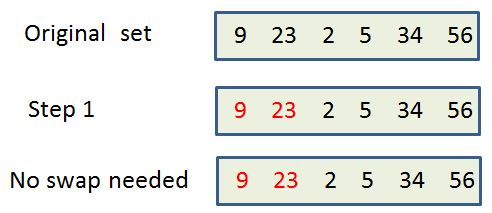Step 2: Move forward by one position and compare the next two numbers in the list - numbers 23 and 2

23 is larger than 2 so the bubble sort will swap the position of those two items.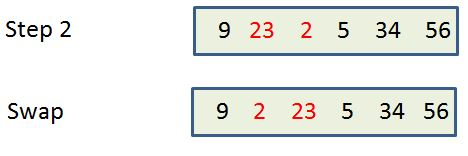Step 3: Move forward by one position and compare the next two numbers in the list - numbers 23 and 5

23 is greater than 5 so they are swapped.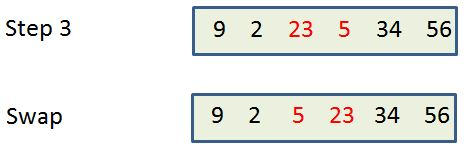Step 4: Move forward by one position and compare the next two numbers in the list - numbers 23 and 34

23 is not greater than 34 so do NOT swap their position.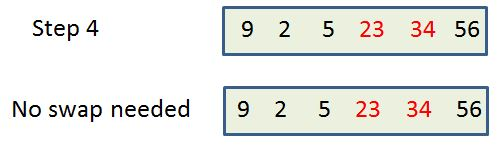Step 5: Move forward by one position and compare the next two numbers in the list - numbers 34 and 56

34 is not greater than 56 so do not swap.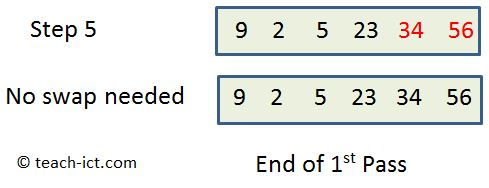This is the end of the first pass.

You can see from the final image above that the data set is not quite sorted in ascending order (the 9 and the 2 are the wrong way around) so the process is repeated once again, starting at the beginning of the list.

The algorithm is complete when it finishes a pass without having to perform any swaps.

Challenge see if you can find out one extra fact on this topic that we haven't already told you

Click on this link: What is bubble sorting?